6000字详细介绍类和对象

# 0. 前言

• ### 阅读本文所需的基础知识

• C语言结构体
• static和const修饰
• 函数的传参
• C++命名空间及作用域
• 面向对象的编程思想

# 1. 类的引入

• ### 从结构体到类

``````struct MyStruct {
int a;
int b;
};
``````

``````struct MyStruct st;
st.a = 1;
st.b = 2;
``````

``````//C
struct MyStruct st;
//C++
MyStruct st;
``````

• ### 类的定义

``````class MyClass {
public:
void function();
private:
int i;
};	//类的定义
``````

`类`类型创建对象的过程，称为类的`实例化`

``````MyClass A;	//类的实例化
``````

• ### 访问限定符

C++使用`访问限定符`限定类中成员的访问，分为以下三种：

public 公有的 在类外可以直接访问
private 私有的 只能被类中的成员函数访问，类外无法直接访问
protected 保护的 能被类中和子类的成员函数访问，类外无法直接访问

``````class MyClass {
public:
void func1() {
cout << "qwq\n";
}
private:
int a;
char str;
public:
static int i;
};
``````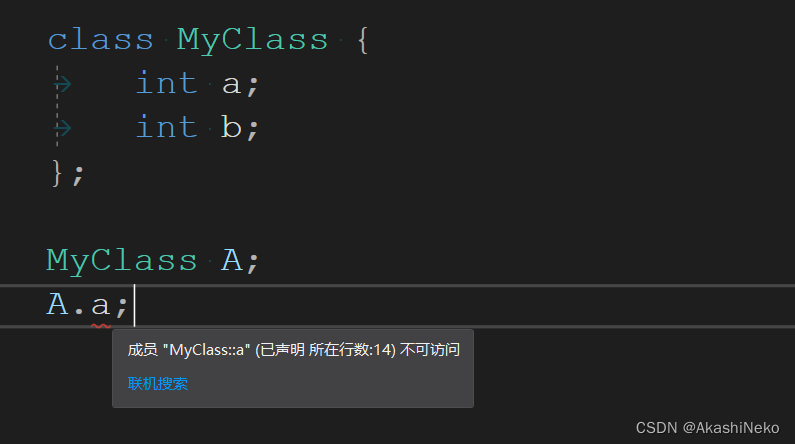• ### 类的作用域

``````class A{
public:
static void fun(){
cout << "qwq\n";
}
};
//在类外调用类A中的公有静态成员函数fun
A::fun();
``````

# 2. 类对象在内存中的储存形式

• ### 计算类对象的大小

``````class A {
public:
void fun1(){
cout << 1;
}
void fun2(){
cout << 2;
}
private:
int a;
int b;
};
``````

`sizeof`计算类的大小和类实例化的对象的大小：

``````A a1;
cout << sizeof(A) << endl;
cout << sizeof(a1) << endl;
``````

``````8
8
``````

• ### 对象在内存中的储存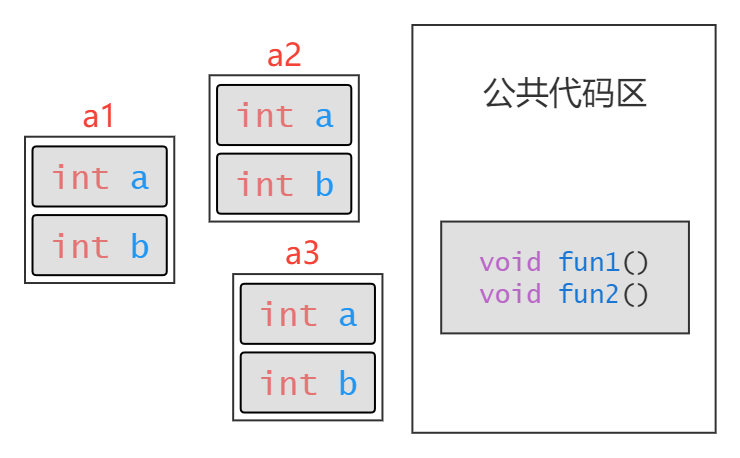事实上，类实例化的对象，只会将类中成员变量按照结构体对齐规则储存，而类中的成员函数则保存在公共代码区，不占用对象的空间，如上图所示。

# 3. 类的成员函数

• ### 成员函数的定义

``````class A {
public:
//类中声明
void fun1();
private:
void fun2();
int _a;
int _b;
};
//类外定义
void A::fun1() {
cout << _a;
}
void A::fun2() {
cout << _b;
}
``````

• ### 成员函数的调用

``````class A {
public:
void fun() {
cout << 1;
}
void init(int a) {
_a = a;
}
private:
int _a;
};
``````
``````int main() {
A a1;
a1.fun();   //调用成员函数fun
a1.init(3); //调用成员函数init
return 0;
}
``````

``````class A {
public:
void print() {
cout << "qwq\n";
}
};

int main() {
A().print();
return 0;
}
``````

• ### 成员函数的传参 - this指针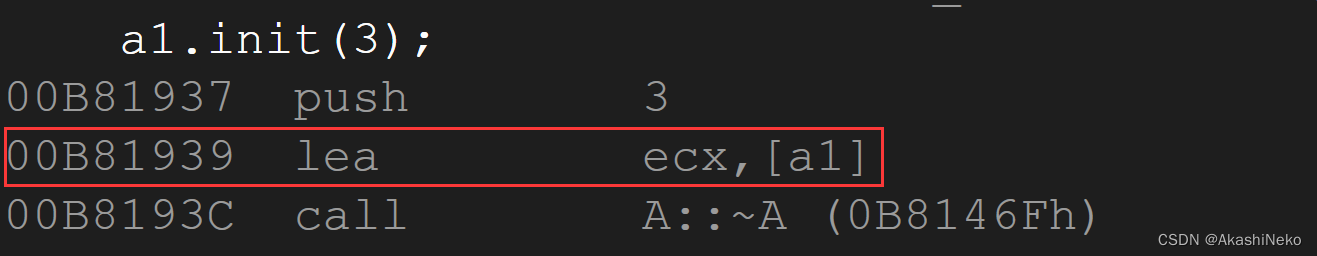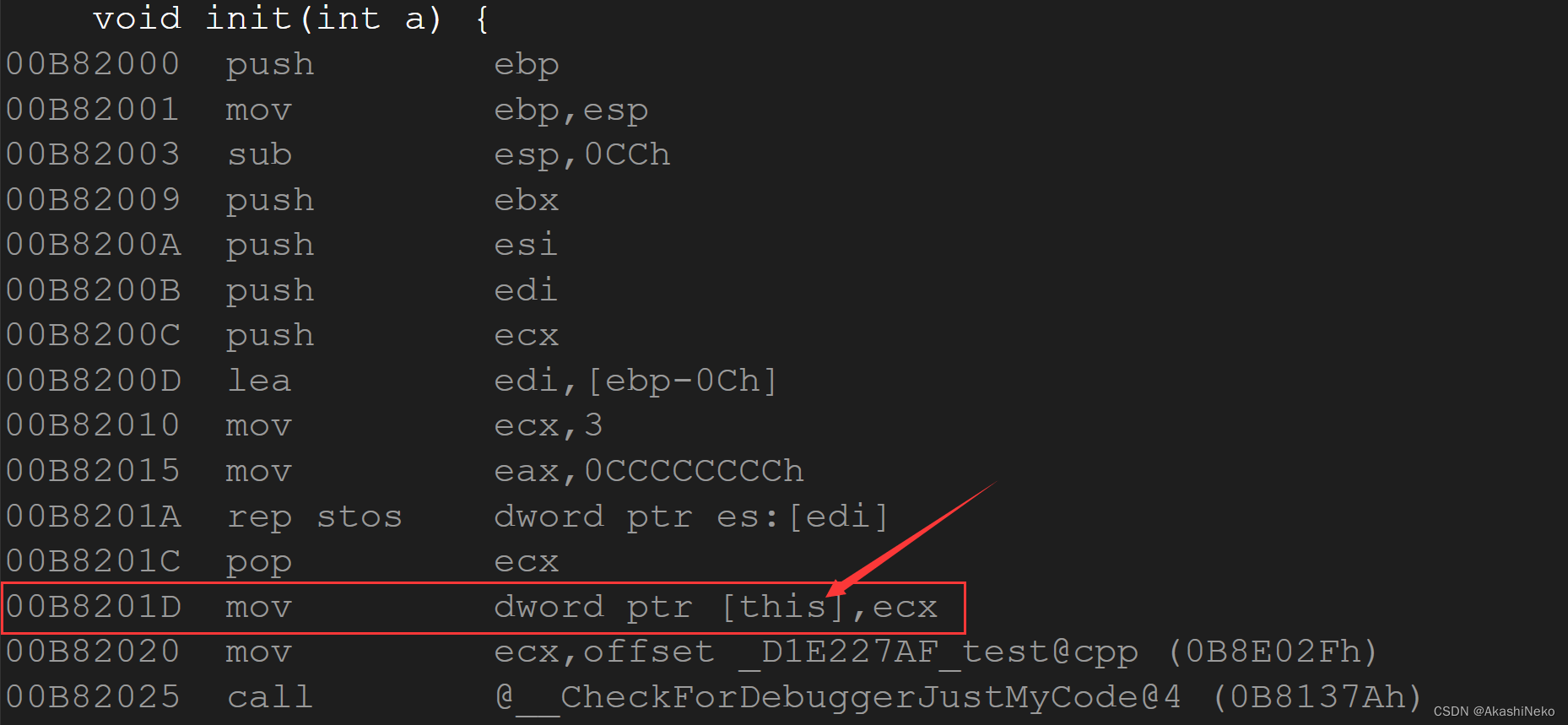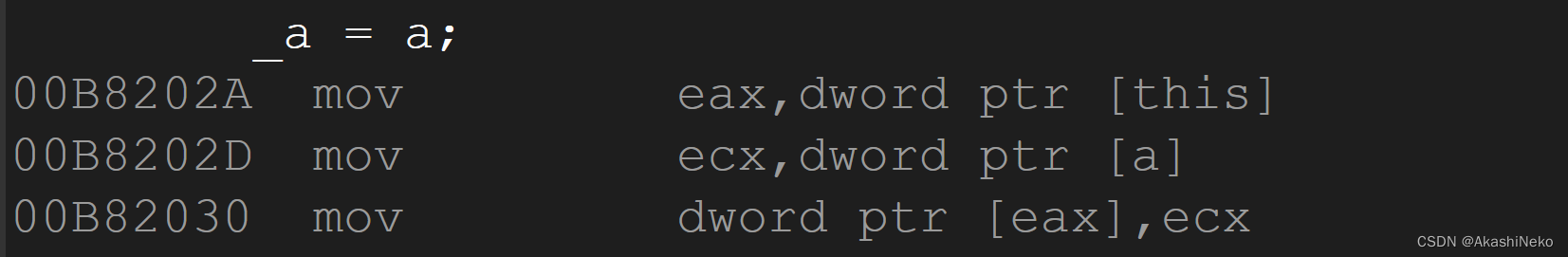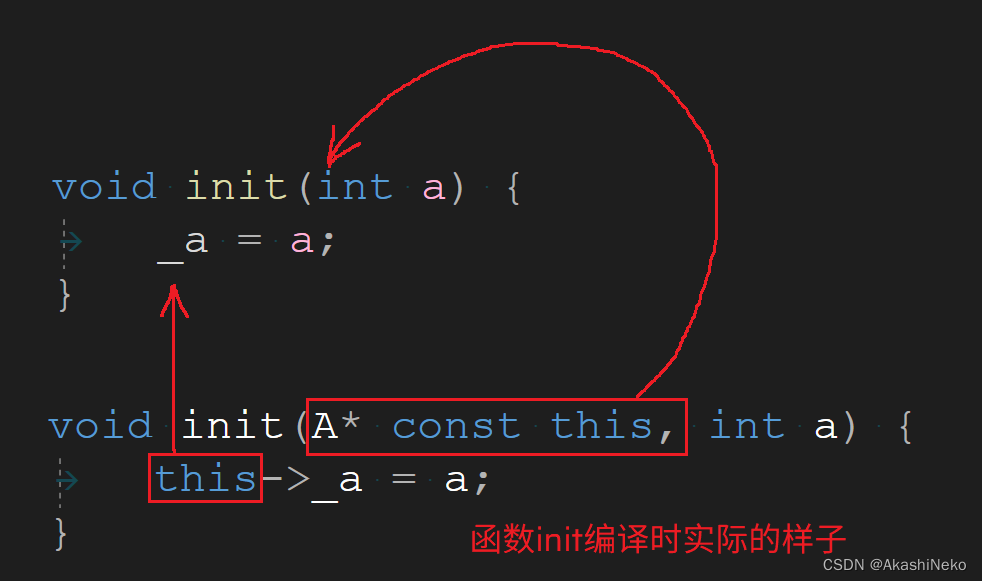• ### const修饰的成员函数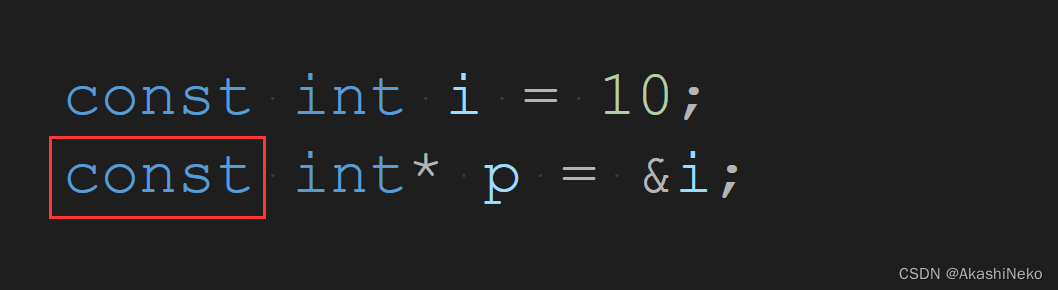``````class A {
public:
void fun() {
//	void fun(A* const this) <-实际的形式
cout << _a << endl;
}
int _a;
};
``````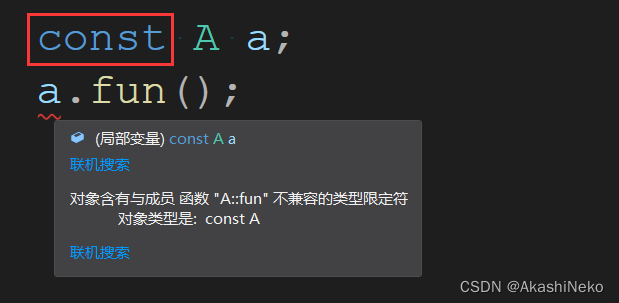``````
class A {
public:
void fun() const {
//	void fun(const A* const this) <-实际的形式
cout << _a << endl;
}
int _a;
};

``````

• ### this指针的使用

`this`为空指针时，只要`this`不发生解引用，程序依然能正常运行：

``````class A {
public:
void print() {
cout << "qwq\n";
}
private:
int _a;
};

int main() {
A* pa = nullptr;
pa->print();
return 0;
}
``````

`this`指针也可以作为返回值使用，观察下面代码中的`add`函数：

``````class A {
public:
void init(int a = 0) {
_a = a;
}
++_a;
return *this;
}
void print() {
cout << _a << endl;
}
private:
int _a;
};
``````
``````int main() {
A a;
a.init(0);
a.print();
a.print();
return 0;
}
``````

``````0
2
``````

# 4. static修饰的成员

• ### 静态成员变量

``````int a = 0; //全局变量 储存在静态区

void fun(){
int a = 10; //局部变量 储存在栈区
int* p = (int*)malloc(sizeof(int));
*p = 20; //在堆中开辟的空间 储存在堆区
static int s = 30; //静态变量 储存在静态区
}
``````

``````//在类中声明静态成员变量
class A {
public:
static int _a;
private:
int _i;
static int _b;
};
``````

``````int A::_a = 10;
int A::_b = 20;
``````

``````class A {
public:
static int _s1;
static int _s2;
int _i;
};

int A::_s1 = 0;
int A::_s2 = 0;

int main() {
A a;
//通过对象可以访问普通成员变量和静态成员变量
a._i = 10;
a._s1 = 20;
//通过类域可以直接访问静态成员变量
A::_s2 = 30;
//	A::_i = 40; //错误的 不能通过类域访问普通成员变量
return 0;
}
``````

• ### 静态成员函数

``````class A {
public:
static void print() {
cout << _a << endl;

//	cout << _i << endl;
//	错误的 静态成员函数没有this指针，无法访问非静态成员变量
}
private:
static int _a;
int _i;
};
``````
``````int A::_a = 1;

int main() {
A::print(); //通过类域直接访问静态成员函数
return 0;
}
``````

`static`成员总结：

# 5. 友元

• ### 友元函数

``````class A {
public:
//声明友元函数
friend void fun(A& a);
private:
int _i;
};

void fun(A& a) {
//在非成员函数中访问类的私有成员变量
a._i = 1;
}
``````

• ### 友元类

``````class A {
public:
friend class B;
private:
int _a;
};

class B {
public:
void fun() {
A a1;
//因为B是A的友元类，所以可以直接访问A中私有的_a成员
a1._a = 1;
}
private:
int _b;
};
``````

``````class A {
public:
friend class B;
private:
int _a;
};

class B {
public:
friend class C;
private:
int _b;
};

class C {
public:
void fun() {
A a1;
//	a1._a = 1;
//  错误的 A和C之间没有友元关系
}
private:
int _c;
};
``````

• ### 内部类

``````class A {
public:
//B定义在A的内部
class B {
public:
void fun() {
cout << _i << endl;
A a1;
//B是A的友元
a1._a = 1;
}
};
private:
int _a;
static int _i;
};
``````